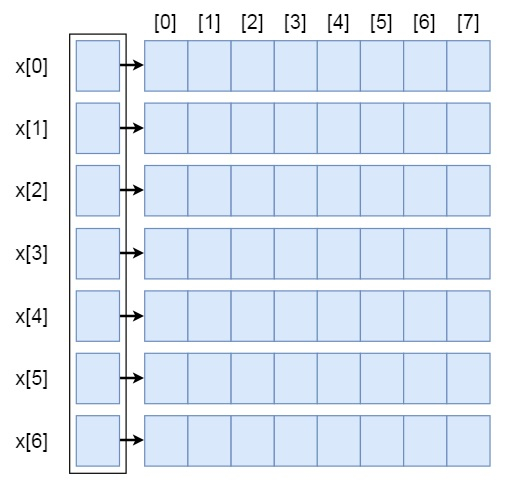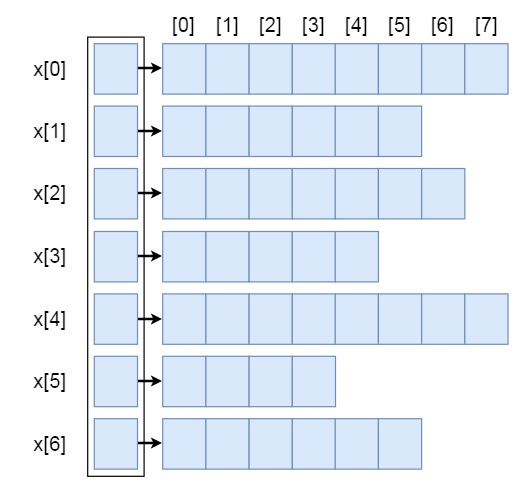# Irregular Arrays in Data Structure

Data StructureAnalysis of AlgorithmsAlgorithms

Here we will see the Irregular arrays. Before discussing the irregular arrays, we have to know what are regular arrays. The regular arrays are that kind of arrays, where the number of columns in each row is same. Or in other words, when each row is holding same number of elements, then that is regular arrays. The following representation is a regular array.From the definition of regular array, we can understand what are the irregular arrays. So in irregular arrays, each row may or may not contain same number of elements. This kind of irregular arrays can also be represented by the Array of Arrays representation. This will be look like below −